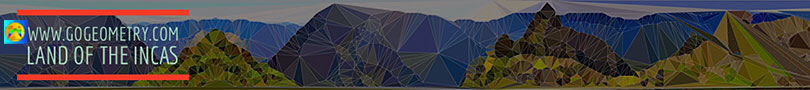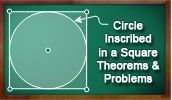# Circle Inscribed in a Square: IndexGeometry Problem 1427.
Circle inscribed in a square, Arc, Tangent Line, Tangent Circles, Radii.

Geometry Problem 1186
Right Triangle, Square, Inscribed Circle, Tangent, Quadrilateral, Area.

Geometry Problem 1184
Right Triangle, Square, Inscribed Circle, Tangent, Concurrent Lines.

Geometry Problem 878
Square, Inscribed Circle, Quadrant, Center, Perpendicular, Metric Relations.

Geometry Problem 697.
Square, Circle, Sector, Segment, Tangent, Inscribed, Congruence, Mind Map, Polya, Circle Inscribed in a Square.

Geometry Problem 693.
Square, Inscribed and Circumscribed Circle, Areas, Mind Map, Polya.

Geometry Problem 692.
Quatrefoil, Four Equal Circles and a Circle Inscribed in a Square, Areas, Mind Map, Polya
.

Geometry Problem 646.
Archimedes Book of Lemmas: Proposition 7
Square,Inscribed and Circumscribed Circles, Area.

Problem 491.
Square, Right Triangle, Incircle, Inscribed Circle, Radius.

Problem 485.
Square, Side, Circles, Tangent, Radius, Measurement, Circle Inscribed in a Square.

Problem 462.
Square, Arcs, 90 Degrees, Circle, Tangent, Radius, Measurement Circle Inscribed in a Square.

Proposed Problem 458.
Square, Semicircle, Circular Sector, Internal Common Tangent, Measurement, Circle Inscribed in a Square.

Proposed Problem 394.
Square, 90 Degree Arc, Diagonal, Congruence, Circle Inscribed in a Square.

Proposed Problem 373.
Square, Inscribed Circle, Diagonal, Perpendicular, Angle.

Proposed Problem 371.
Square, Inscribed circle, Triangle, Area.

Proposed Problem 331.
Square, Point on the Inscribed Circle, Tangency Points.

Proposed Problem 322.
Square, Inscribed circle, Tangent, Triangle area.

Archimedes' Book of Lemmas: Proposition 7
Square and inscribed and circumscribed Circles

Proposed Problem 276.
Square, 90 degree Arcs, Circle, Radius.

Problem 112. Area of square and triangle. Circle Inscribed in a Square.

Problem 76: Area of a Circle. Circle Inscribed in a Square, Circular Sector.

Problem 62: Square Diagonal, Circle Inscribed in a Square.

 Home | Search | Geometry | Circles | Square | Post a comment | Email | by Antonio Gutierrez Last updated: Jun 3, 2023